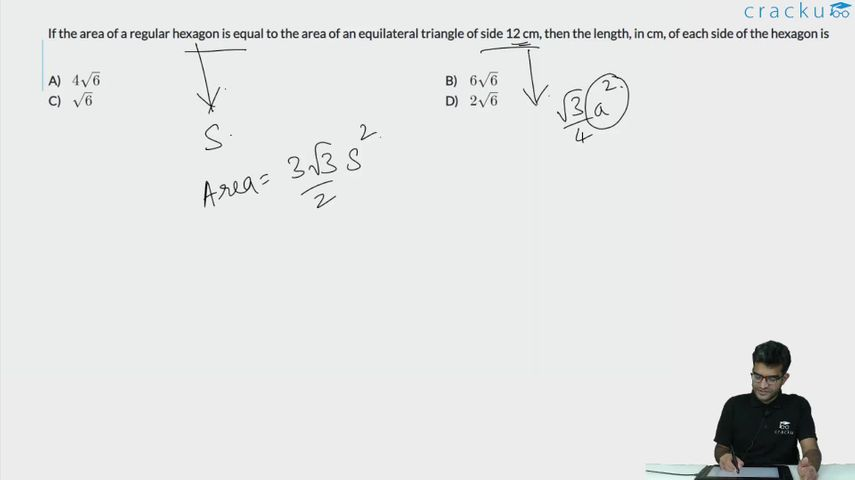Question 2

# If the area of a regular hexagon is equal to the area of an equilateral triangle of side 12 cm, then the length, in cm, of each side of the hexagon is

Solution

Area of a regular hexagon = $$\frac{3\sqrt{3}}{2}x^2$$

Area of an equilateral triangle = $$\frac{\sqrt{3}}{4}\left(a\right)^2$$ ; where a = side of the triangle

Since the area of the two figures are equal, we can equate them as folllows: $$\frac{3\sqrt{3}}{2}x^2=\frac{\sqrt{3}}{4}\left(12\right)^2$$

On simplifying: $$x^2=24\$$

$$\therefore\ x=2\sqrt{6}$$

### View Video Solution